APPLIED INDUSTRIAL CONTROL SOLUTIONS LLCAudio Stereo Speaker Cabinet Design Calculator, a Tutorial PART 1

Stereo Driver/Speaker Cabinet Matching Equations

You may have purchased stereo drivers and when looking at the manufacturers published specs you feel that the values don't seem correct. Maybe you purchased an inexpensive driver for testing purposes and had no specs at all. You will find here the methods and formulas to determine the parameters needed to design boxes and filters.

When wanting to measure the parameters of any transducer, a minimum of instruments are required for the task. You will need a multimeter with voltage and Ohms measurement capabilities. You will also need a frequency generator that is variable from about 20Hz to 20KHz. A stereo linear Amplifier. Other accessories include resistors one of 1000 Ohms and one of 10 Ohms. These instruments are the minimun required to determine any usefull parameters.

Microphones and anechoic chambers will not be discussed.

Before performing any mesurements, any driver should be broken in (played at moderately high volume for an extended period) prior to these mesurements as the parameters change once broken in.

Re
DC resistance is determined with an Ohms meter connected to it's terminals.

Le
Le is mesured using a small setup consisting of every item in the instruments list, here is the setup diagram.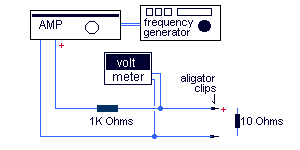The 10 Ohms resistor is used to calibrate the setup so it should be a very precise and linear resistor.
With the resistor connected to the end connectors or aligator clips, set the frequency generator at approximatly 1000 Hertz. Ajust the gain on the Amplifier and the frequency generator until the AC voltmeter reads 10 volts. If you feel your amplifier or any component in your setup is not as linear as it should, reajust the gain with the resistor at every frequecy you will use to make your readings.
To find the Le you must set the frequency at the drivers highest usable frequency. By removing the 10 Ohms resistor and placing the driver at its place. The reading on the voltmeter will be what's called the driver's impedance magnitude (M) for this frequency. It is then used in this formula to find Le .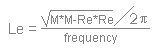You can also find Le with the following calculator using the values you have found with the setup.
 Inductance = mH DC Resistance Ohms Frequency Hz Mesured Impedance at frequency Ohms

Ce
The value of Ce is determined with Re and Le with the following formula.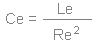You can also find Ce with the following calculator using Re and Le.
 Capacitance = uF DC Resistance Ohms Inductance mH

Fs
Finding the value of fs is quite simple. Simply note the frequency at the apex of the impedance peak(Zmax).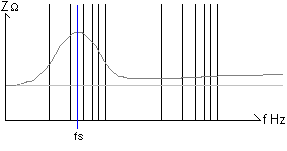Qms
To find Qms, the formula requires you to find the value of R0 first. R0 is the value of Zmax at fs over Re.Then the product of Re and the square root of R0 will give you the value of R1.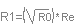Then above and below fs, note the frequencies where the impedance is equal to R1 Those values will be f1 and f2 as in the following diagram.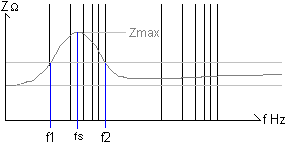Qms is then found with the following formula.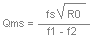Qes
Qes is found with the following formula using Qms and R0.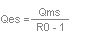Qts
Qts is found with the following formula using Qms and Qes.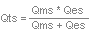Mmd
To find the moving mass of the driver you will first need to know the fs of the driver as shown above. You will then need a calibrated weight(M') to be attached on the driver's cone, usually a piece of clay. Once the weight(M') is firmly attached to the cone find the driver's new fs(fs').
You can then find Mmd with the following formula;
Mmd = M' / (fs/fs')2 - 1

Cms
Cms requires fs and Mmd in the following formula;
Cms = 1 / (2pfs)2 * Mmd

Sd
The driver's effective piston Area is foud with the following formula.
Sd = pr2
Where "r" is the radius of the cone.

Vas
By taking great care in finding Cms you can find Vas with the former with this formula.
Note that Sd must be in centimeters.
Vas = Cms * De * Cs2 * Sd2
In the preceding formula "De" represents the density of air in grams per cubic centimeter, tipically 0.0013 grams per cubic centimeter. "Cs" is the speed of sound in air, here in centimeters per second. Under normal circumstaces, at audible frequencies, this value is about 34400 centimeters per second.